# 5 Trigonometric Identities 5 1 Fundamental Identities Using

• Slides: 245 Trigonometric Identities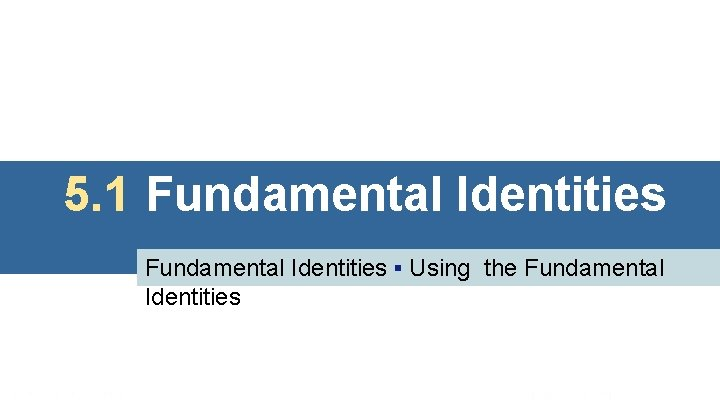5. 1 Fundamental Identities ▪ Using the Fundamental IdentitiesFundamental Identities An identity is an equation that is satisfied by every value in the domain of its variable.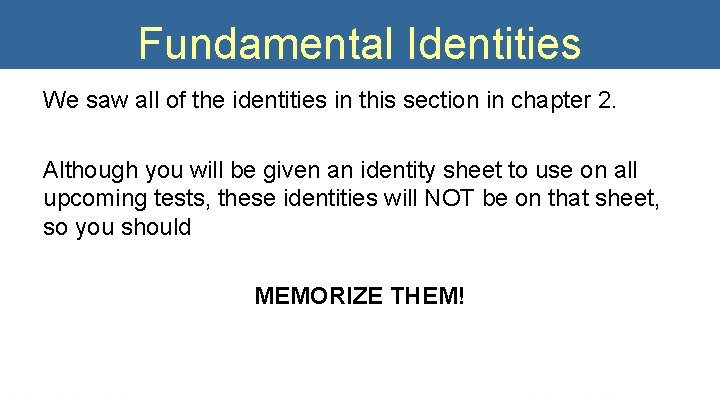Fundamental Identities We saw all of the identities in this section in chapter 2. Although you will be given an identity sheet to use on all upcoming tests, these identities will NOT be on that sheet, so you should MEMORIZE THEM!Negative-Angle Identities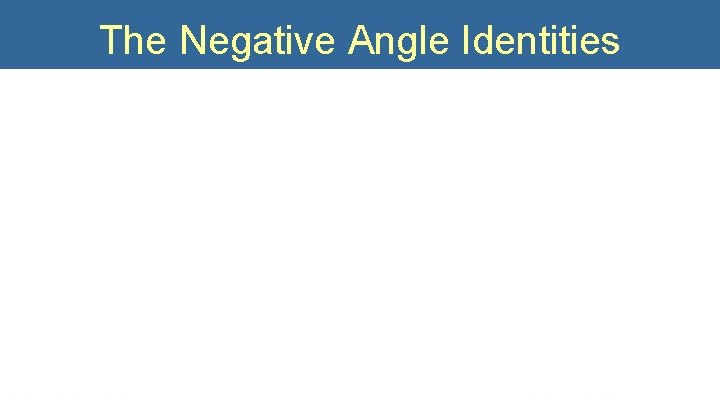The Negative Angle Identities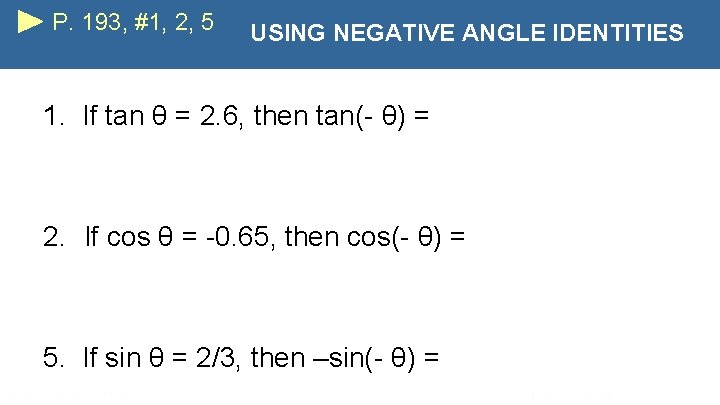P. 193, #1, 2, 5 USING NEGATIVE ANGLE IDENTITIES 1. If tan θ = 2. 6, then tan(- θ) = 2. If cos θ = -0. 65, then cos(- θ) = 5. If sin θ = 2/3, then –sin(- θ) =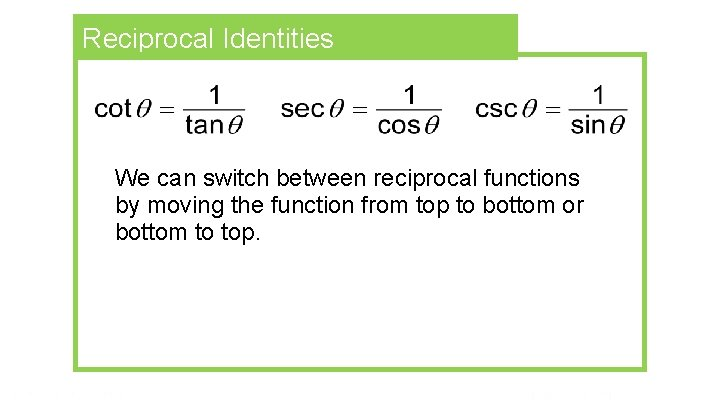Reciprocal Identities We can switch between reciprocal functions by moving the function from top to bottom or bottom to top.P. 195, #46, 63 USING RECIPROCAL IDENTITIES Write in terms of sine and cosine and simplify so that no quotients appear. 46. 63. cos θ csc θQuotient Identities For all angles θ for which the denominators are not zero, the following identities hold.Deriving the Quotient Identities r=1 θPythagorean Identities For all angles θ for which the function values are defined, the following identities hold.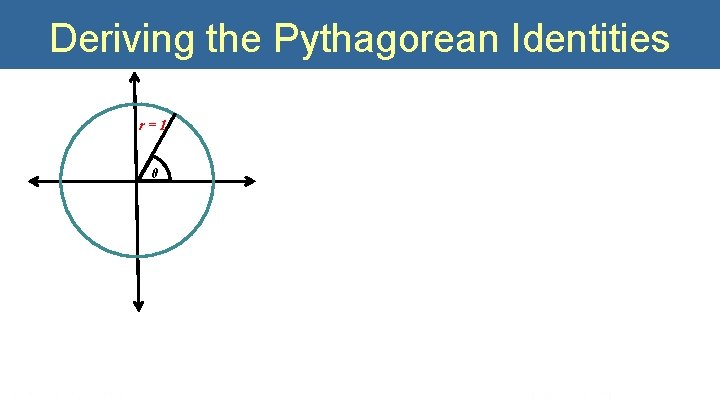Deriving the Pythagorean Identities r=1 θ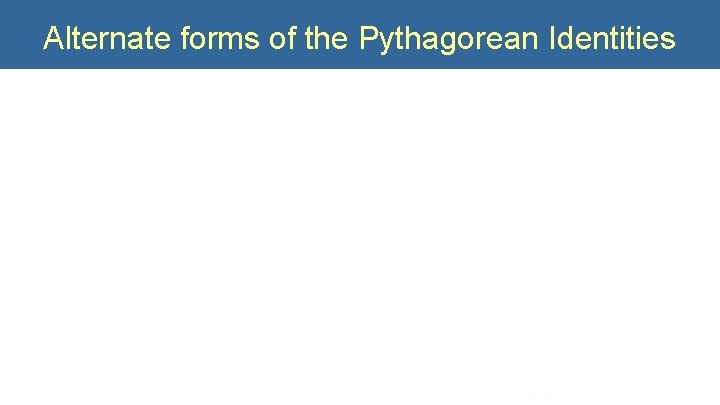Alternate forms of the Pythagorean Identities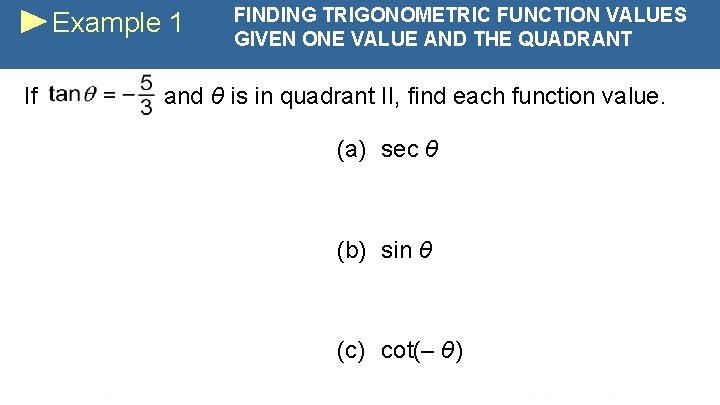Example 1 If FINDING TRIGONOMETRIC FUNCTION VALUES GIVEN ONE VALUE AND THE QUADRANT and θ is in quadrant II, find each function value. (a) sec θ (b) sin θ (c) cot(– θ)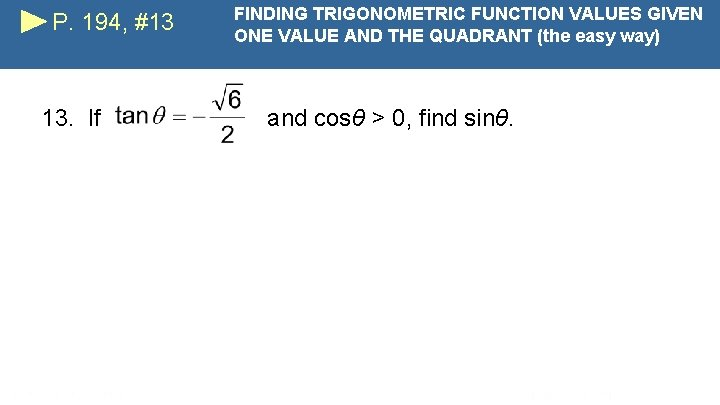P. 194, #13 13. If FINDING TRIGONOMETRIC FUNCTION VALUES GIVEN ONE VALUE AND THE QUADRANT (the easy way) and cosθ > 0, find sinθ.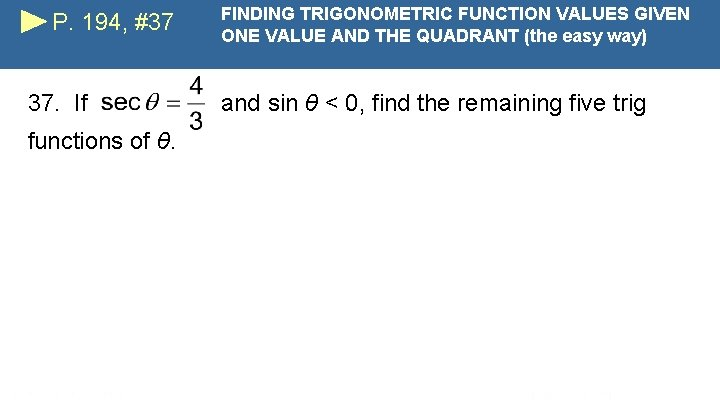P. 194, #37 37. If functions of θ. FINDING TRIGONOMETRIC FUNCTION VALUES GIVEN ONE VALUE AND THE QUADRANT (the easy way) and sin θ < 0, find the remaining five trig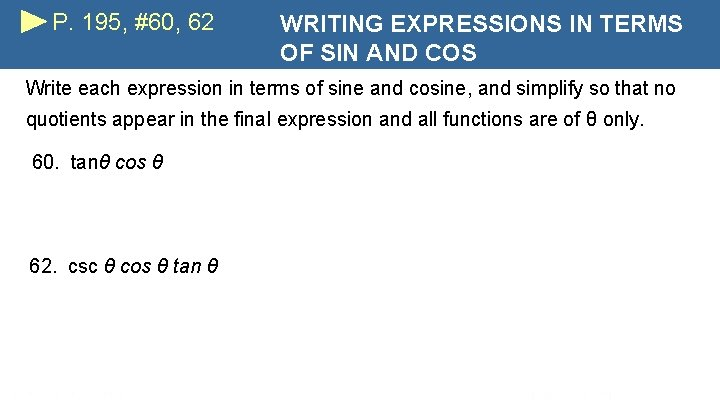P. 195, #60, 62 WRITING EXPRESSIONS IN TERMS OF SIN AND COS Write each expression in terms of sine and cosine, and simplify so that no quotients appear in the final expression and all functions are of θ only. 60. tanθ cos θ 62. csc θ cos θ tan θP. 195, #76 WRITING EXPRESSIONS IN TERMS OF SIN AND COS Write each expression in terms of sine and cosine, and simplify so that no quotients appear in the final expression and all functions are of θ only. 76. (sin θ - cos θ)(csc θ + sec θ)P. 195, #80 PYTHAGOREAN SUBSTITUTION Write each expression in terms of sine and cosine, and simplify so that no quotients appear in the final expression and all functions are of θ only. 80.Example 3 Write REWRITING AN EXPRESSION IN TERMS OF SINE AND COSINE in terms of sin θ and cos θ, and then simplify the expression so that no quotients appear.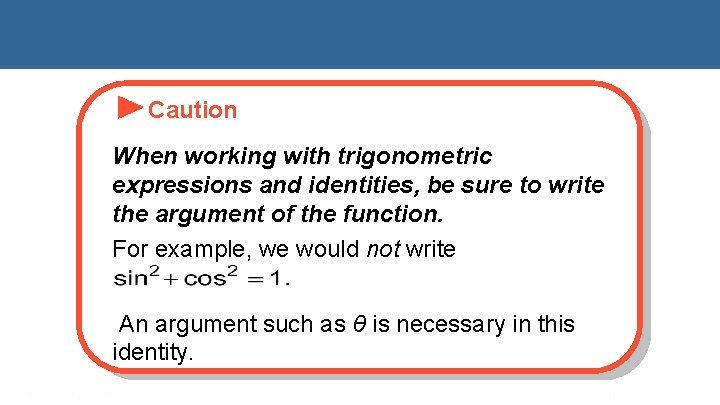Caution When working with trigonometric expressions and identities, be sure to write the argument of the function. For example, we would not write An argument such as θ is necessary in this identity.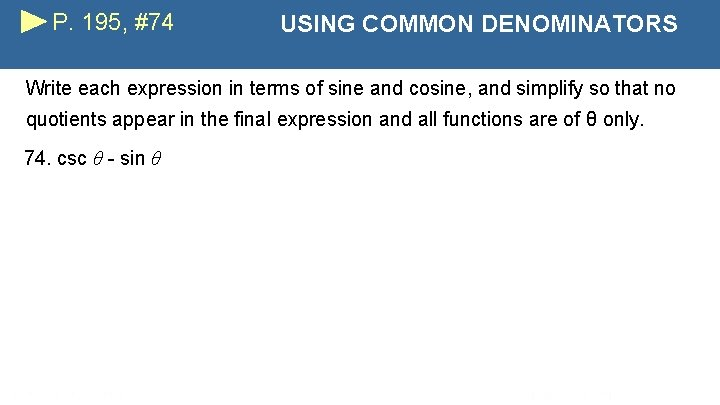P. 195, #74 USING COMMON DENOMINATORS Write each expression in terms of sine and cosine, and simplify so that no quotients appear in the final expression and all functions are of θ only. 74. csc θ - sin θP. 195, #69 SPLITTING ONE FRACTION INTO TWO FRACTIONS Write each expression in terms of sine and cosine, and simplify so that no quotients appear in the final expression and all functions are of θ only. 69.